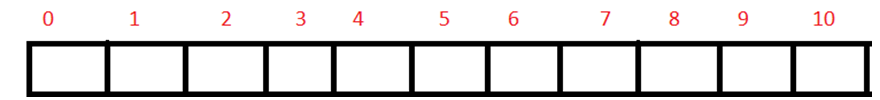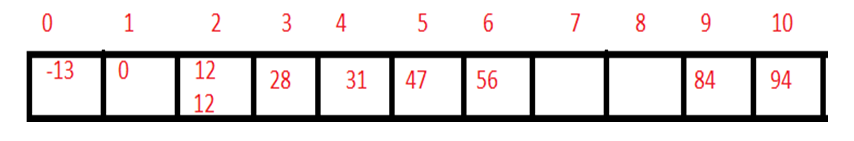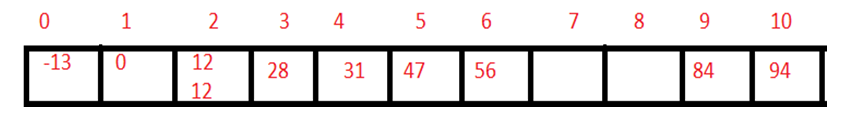# Bucket Sort Algorithm

In this article, we will learn about Bucket sort with its algorithm and pseudo code. Examples of bucket sort with its analysis are also prescribed in this article.
Submitted by Abhishek Kataria, on July 18, 2018

## Bucket Sort

Bucket sort is a sorting technique in which array is partitioned into the buckets. By this, each bucket will be sorted individually, by using some another sorting algorithm such as insertion sort. This sorting technique assumes that the input is drawn from a uniform distribution by which it has an average case of O(n). Bucket sort is a fast technique because bucket sort presumes that the input is generated by a process, which will distribute the element uniformly and independently over the interval.

## Algorithm for Bucket Sort

1. Set up an array of initially empty buckets.
2. Put the element in the corresponding bucket.
3. Sort each non-empty bucket.
4. Visit the bucket in order and put all the elements into a sequence and print them.

### Pseudo code for Bucket Sort

```void bucketsort (int a[ ], int n, int max)
{
int i,j=0;
//initialize each bucket 0 and then make bucket empty.
int* buckets = calloc(max+1, size of (int));
for(int i=0; i<n; i++)
buckets[a[i]]++;
//now sort each bucket individually.
//sequentially empty each bucket in some array.
for(i=0; i<max; i++)
while (buckets[i]--)
b[j++]=i;
//display the array b as sorted list of elements.
}
```

Example:

Let us sort the elements by using bucket sort. Elements which need to be sort are 56, 12, 84, 28, 0,-13, 47, 94, 31, 12.

Step 1) First set up an array which is given below:Step 2) Now consider the range such that:

-20 to -1, 0 to 10 10 to 20, 20 to 30, 30 to 40, 40 to 50, 50 to 60, 60 to 70, 70 to 80, 80 to 90, 90 to 100.

Now we fill up each bucket by given elements,Step 3) Now sort the each bucket and then print the array by visiting each bucket sequentially.-13, 0, 12, 12, 28, 31, 47, 56, 84, 94

This is the sorted list.

### Drawbacks of Bucket Sort

1. For the bucket sort, it’s the necessary part that the maximum value of the element must be known.
2. In this type of technique, we have to create enough buckets in the memory for every element, to place in the array.

### Analysis of the bucket Sort

Average case, best case, and worst case time complexity of this algorithm is O(n).

What's New (MCQs)

Top Interview Coding Problems/Challenges!

Languages: » C » C++ » C++ STL » Java » Data Structure » C#.Net » Android » Kotlin » SQL
Web Technologies: » PHP » Python » JavaScript » CSS » Ajax » Node.js » Web programming/HTML
Solved programs: » C » C++ » DS » Java » C#
Aptitude que. & ans.: » C » C++ » Java » DBMS
Interview que. & ans.: » C » Embedded C » Java » SEO » HR
CS Subjects: » CS Basics » O.S. » Networks » DBMS » Embedded Systems » Cloud Computing
» Machine learning » CS Organizations » Linux » DOS
More: » Articles » Puzzles » News/Updates

© https://www.includehelp.com some rights reserved.